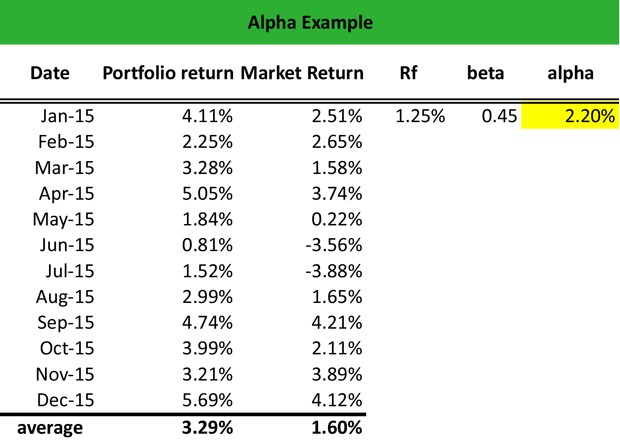# What is Alpha?

Definition: Alpha, also known as Jensen Index, is a measure of portfolio performance under the condition that a portfolio is well-diversified to leverage unsystematic risk.

## What Does Alpha Mean?

What is the definition of alpha? Financial analysts use alpha when they seek to determine the highest possible return on investment with the lowest level of risk. Therefore, the main assumption of alpha is that a portfolio is adequately diversified to generate a high return while leveraging the portfolio risk.

Also, as alpha represents the portfolio performance, it is always evaluated in comparison to a benchmark, under the assumption that the portfolio’s return on investment is not related to the market like the beta (systematic risk), but to the individual selections of the fund manager. Hence, an alpha equal to zero simply means that the portfolio is perfectly correlated to the benchmark index.

Let’s look at an example.

## Example

Marion is a fund manager at Morgan Stanley, and she handles the portfolios of several clients. All portfolios are well-diversified, including large cap equity, small cap equity, emerging markets equities, bonds, and T-bills. Marion wants to calculate the alpha of a portfolio that is underperforming the market over the past few months to determine the highest possible return on investment at the lowest level of risk.

Hence, Marion uses the capital price asset model (CAPM) to calculate alpha:

alpha = rs – [Rf + b (Rm – Rf)]

where:

Marion calculates the average portfolio return and the average market return for 2015, and she finds that the portfolio return (rs) is 3.29%, whereas the market return (rm) is 1.60%. The risk-free rate (rf) is 1.25%.

Marion can now calculate the portfolio beta and the portfolio alpha as follows:

In Excel, beta is calculated using the covariance. p function and the variance. p function. Therefore, beta = (covariance. p of the portfolio return and the market return cells) /variance. p (market return). Doing these calculations, Marion finds that the portfolio beta is 0.45.

Then, she calculates alpha by applying the above formula. Therefore:Given the low beta of the portfolio, the positive alpha of 2.20% suggests that Marion earns more than a sufficient return given the level of risk undertaken in this portfolio.

## Summary Definition

Define Alpha: Alpha means a financial performance metric that investors use to gauge their portfolio performance.